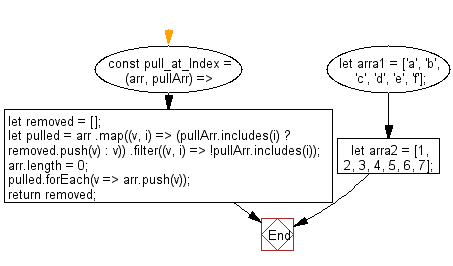# JavaScript: Extract out the values at the specified indexes from a specified array

## JavaScript fundamental (ES6 Syntax): Exercise-10 with Solution

Write a JavaScript program to extract out the values at the specified indexes from a specified array.

• Use Array.prototype.filter() and Array.prototype.includes() to pull out the values that are not needed.
• Set Array.prototype.length to mutate the passed in an array by resetting its length to 0.
• Use Array.prototype.push() to re-populate it with only the pulled values.
• Use Array.prototype.push() to keep track of pulled values.

Sample Solution:

JavaScript Code:

``````//#Source https://bit.ly/2neWfJ2
const pull_at_Index = (arr, pullArr) => {
let removed = [];
let pulled = arr
.map((v, i) => (pullArr.includes(i) ? removed.push(v) : v))
.filter((v, i) => !pullArr.includes(i));
arr.length = 0;
pulled.forEach(v => arr.push(v));
return removed;
};
let arra1 = ['a', 'b', 'c', 'd', 'e', 'f'];
console.log(pull_at_Index(arra1, [1, 3]));
let arra2 =  [1, 2, 3, 4, 5, 6, 7];
console.log(pull_at_Index(arra2, ));
```
```

Sample Output:

```["b","d"]

```

Pictorial Presentation:Flowchart:Live Demo:

See the Pen javascript-basic-exercise-1-10 by w3resource (@w3resource) on CodePen.

Improve this sample solution and post your code through Disqus

What is the difficulty level of this exercise?

Test your Programming skills with w3resource's quiz.

﻿

## JavaScript: Tips of the Day

Returns an array of n-tuples of consecutive elements

Example:

```const tips_arr = (n, arr) =>  n > arr.length ? [] : arr.slice(n - 1).map((v, i) =>[...arr.slice(i, i + n - 1), v]);
console.log(tips_arr(2, [1, 2, 3, 4, 5]));
console.log(tips_arr(3, [1, 2, 3, 4, 5]));
console.log(tips_arr(5, [1, 2, 3, 4]));
```

Output:

```[[1, 2], [2, 3], [3, 4], [4, 5]]
[[1, 2, 3], [2, 3, 4], [3, 4, 5]]
[]
```